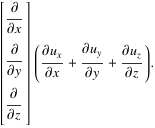## Sunday, May 24, 2009

### [Mathematic Form 4] Cartesian Coordinates

Cartesian coordinates are rectilinear two-dimensional or three-dimensional coordinates (and therefore a special case of curvilinear coordinates) which are also called rectangular coordinates. The three axes of three-dimensional Cartesian coordinates, conventionally denoted the x-, y-, and z-axes (a notation due to Descartes) are chosen to be linear and mutually perpendicular. In three dimensions, the coordinates x, y, and z may lie anywhere in the interval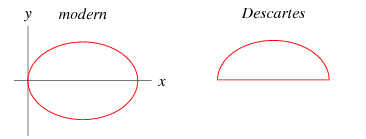In René Descartes' original treatise (1637), which introduced the use of coordinates for describing plane curves, the axes were omitted, and only positive values of the x- and the y-coordinates were considered, since they were defined as distances between points. For an ellipse this meant that, instead of the full picture which we would plot nowadays (left figure), Descartes drew only the upper half (right figure).

The inversion of three-dimensional Cartesian coordinates is called 6-sphere coordinates.

The scale factors of Cartesian coordinates are all unity, h_i=1. The line element is given by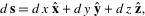and the volume element byThe gradient has a particularly simple form,as does the LaplacianThe vector Laplacian,, isThe divergence isand the curl,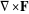, is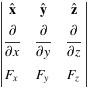The gradient of the divergence,, is